# 五种常见的 PHP 设计模式

+关注继续查看

## 工厂模式

##### 清单 1. Factory1.php
<?php
interface IUser
{
function getName();
}

class User implements IUser
{
public function __construct( $id ) { } public function getName() { return "Jack"; } } class UserFactory { public static function Create($id )
{
return new User( $id ); } }$uo = UserFactory::Create( 1 );
echo( $uo->getName()."\n" ); ?> IUser 接口定义用户对象应执行什么操作。IUser 的实现称为 UserUserFactory 工厂类则创建 IUser 对象。此关系可以用图 1 中的 UML 表示。 ##### 图 1. 工厂类及其相关 IUser 接口和用户类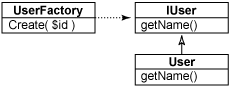如果您使用 php 解释器在命令行上运行此代码，将得到如下结果： % php factory1.php Jack % 测试代码会向工厂请求 User 对象，并输出 getName 方法的结果。 有一种工厂模式的变体使用工厂方法。类中的这些公共静态方法构造该类型的对象。如果创建此类型的对象非常重要，此方法非常有用。例如，假设您需要先创建对象，然后设置许多属性。此版本的工厂模式会将该进程封装在单个位置中，这样，不用复制复杂的初始化代码，也不必将复制好的代码在在代码库中到处粘贴。 清单 2 显示使用工厂方法的一个示例。 ##### 清单 2. Factory2.php <?php interface IUser { function getName(); } class User implements IUser { public static function Load($id )
{
return new User( $id ); } public static function Create( ) { return new User( null ); } public function __construct($id ) { }

public function getName()
{
return "Jack";
}
}

$uo = User::Load( 1 ); echo($uo->getName()."\n" );
?>

##### 图 2. IUser 接口和带有工厂方法的 user 类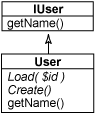% php factory2.php
Jack
%

## 单元素模式

##### 清单 3. Singleton.php
<?php
require_once("DB.php");

class DatabaseConnection
{
public static function get()
{
static $db = null; if ($db == null )
$db = new DatabaseConnection(); return$db;
}

private $_handle = null; private function __construct() {$dsn = 'mysql://root:password@localhost/photos';
$this->_handle =& DB::Connect($dsn, array() );
}

public function handle()
{
return $this->_handle; } } print( "Handle = ".DatabaseConnection::get()->handle()."\n" ); print( "Handle = ".DatabaseConnection::get()->handle()."\n" ); ?> 此代码显示名为 DatabaseConnection 的单个类。您不能创建自已的 DatabaseConnection，因为构造函数是专用的。但使用静态 get 方法，您可以获得且仅获得一个 DatabaseConnection 对象。此代码的 UML 如图 3 所示。 ##### 图 3. 数据库连接单元素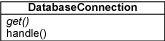在两次调用间，handle 方法返回的数据库句柄是相同的，这就是最好的证明。您可以在命令行中运行代码来观察这一点。 % php singleton.php Handle = Object id #3 Handle = Object id #3 % 返回的两个句柄是同一对象。如果您在整个应用程序中使用数据库连接单元素，那么就可以在任何地方重用同一句柄。 您可以使用全局变量存储数据库句柄，但是，该方法仅适用于较小的应用程序。在较大的应用程序中，应避免使用全局变量，并使用对象和方法访问资源。 ## 观察者模式 观察者模式为您提供了避免组件之间紧密耦合的另一种方法。该模式非常简单：一个对象通过添加一个方法（该方法允许另一个对象，即观察者注册自己）使本身变得可观察。当可观察的对象更改时，它会将消息发送到已注册的观察者。这些观察者使用该信息执行的操作与可观察的对象无关。结果是对象可以相互对话，而不必了解原因。 一个简单示例是系统中的用户列表。清单 4 中的代码显示一个用户列表，添加用户时，它将发送出一条消息。添加用户时，通过发送消息的日志观察者可以观察此列表。 ##### 清单 4. Observer.php <?php interface IObserver { function onChanged($sender, $args ); } interface IObservable { function addObserver($observer );
}

class UserList implements IObservable
{
private $_observers = array(); public function addCustomer($name )
{
foreach( $this->_observers as$obs )
$obs->onChanged($this, $name ); } public function addObserver($observer )
{
$this->_observers []=$observer;
}
}

class UserListLogger implements IObserver
{
public function onChanged( $sender,$args )
{
echo( "'$args' added to user list\n" ); } }$ul = new UserList();
$ul->addObserver( new UserListLogger() );$ul->addCustomer( "Jack" );
?>

##### 图 4. 可观察的用户列表和用户列表事件日志程序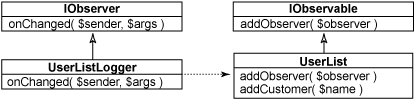% php observer.php
%

## 命令链模式

##### 清单 5. Chain.php
<?php
interface ICommand
{
function onCommand( $name,$args );
}

class CommandChain
{
private $_commands = array(); public function addCommand($cmd )
{
$this->_commands []=$cmd;
}

public function runCommand( $name,$args )
{
foreach( $this->_commands as$cmd )
{
if ( $cmd->onCommand($name, $args ) ) return; } } } class UserCommand implements ICommand { public function onCommand($name, $args ) { if ($name != 'addUser' ) return false;
return true;
}
}

class MailCommand implements ICommand
{
public function onCommand( $name,$args )
{
if ( $name != 'mail' ) return false; echo( "MailCommand handling 'mail'\n" ); return true; } }$cc = new CommandChain();
$cc->addCommand( new UserCommand() );$cc->addCommand( new MailCommand() );
$cc->runCommand( 'addUser', null );$cc->runCommand( 'mail', null );
?>

##### 图 5. 命令链及其相关命令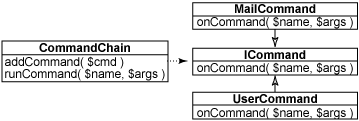% php chain.php
MailCommand handling 'mail'
%

## 策略模式

##### 清单 6. Strategy.php
<?php
interface IStrategy
{
function filter( $record ); } class FindAfterStrategy implements IStrategy { private$_name;

public function __construct( $name ) {$this->_name = $name; } public function filter($record )
{
return strcmp( $this->_name,$record ) <= 0;
}
}

class RandomStrategy implements IStrategy
{
public function filter( $record ) { return rand( 0, 1 ) >= 0.5; } } class UserList { private$_list = array();

public function __construct( $names ) { if ($names != null )
{
foreach( $names as$name )
{
$this->_list []=$name;
}
}
}

public function add( $name ) {$this->_list []= $name; } public function find($filter )
{
$recs = array(); foreach($this->_list as $user ) { if ($filter->filter( $user ) )$recs []= $user; } return$recs;
}
}

$ul = new UserList( array( "Andy", "Jack", "Lori", "Megan" ) );$f1 = $ul->find( new FindAfterStrategy( "J" ) ); print_r($f1 );

$f2 =$ul->find( new RandomStrategy() );
print_r( \$f2 );
?>

##### 图 6. 用户列表和用于选择用户的策略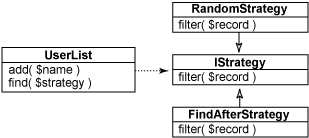UserList 类是打包名称数组的一个包装器。它实现 find 方法，该方法利用几个策略之一来选择这些名称的子集。这些策略由 IStrategy接口定义，该接口有两个实现：一个随机选择用户，另一个根据指定名称选择其后的所有名称。运行测试代码时，将得到以下输出：

% php strategy.php
Array
(
 => Jack
 => Lori
 => Megan
)
Array
(
 => Andy
 => Megan
)
%

## 结束语PHP常用设计模式
PHP常用设计模式
0 0php设计模式-工厂模式
php设计模式-工厂模式
0 0php设计模式-注册器模式
php设计模式-注册器模式
0 0php设计模式-单例模式
php设计模式-单例模式
0 0【PHP-设计模式】职责链模式
【PHP-设计模式】职责链模式
0 0【PHP-设计模式】观察者模式
【PHP-设计模式】观察者模式
0 0PHP设计模式（3）观察者模式
952 0PHP设计模式：观察者模式
639 0

PHP进阶教程 - 由浅入深掌握面向对象开发 - 第一阶段20432

PHP进阶教程 - 由浅入深掌握面向对象开发 - 第二阶段13933

PHP进阶教程 - 由浅入深掌握面向对象开发 - 第三阶段20931

PHP完全自学手册文档教程941088

PHP安全开发_从白帽角度做安全

PHP在机器学习上的应用及云深度学习平台的架构设计与实现

PHP与APM_技术内幕和最佳实践